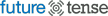# Math Teacher Responds to Slate Article That Criticized Tech and Calculators in the Classroom

Konstantin Kakaes’ article “Why Johnny Can’t Add Without A Calculator,” published on Slate on Monday, is the latest in a long tradition of blaming poor math achievement of U.S. children on the use of calculators and computers in classrooms. In its essence, Kakaes’ argument is:

1. Many math teachers who use technology do so ineffectively.
2. Many students who are taught mathematics with calculators don’t have a good grasp of basic arithmetic, or other “traditional” mathematics.
3. A few teachers teach math successfully without calculators to some students, where “successfully” is defined as “according to traditional criteria.”
4. Therefore, the introduction of calculators and other high-tech tools in math classrooms is to blame for the decline of U.S. math students.

Not only is his logic flawed, but the most important premise—that U.S. math students are doing worse and worse—is not even true.

That students in the United States are behind those in other countries, and doing worse than ever, has been repeated so many times that many take it as fact. But the data suggest otherwise. The other major international study, Trends in Mathematics and Science Study, shows a rise of 11 points (from 518 to 529) in scores of U.S. fourth grade students between 1995 and 2007. During the same period, eighth graders’ scores improved from 492 to 508. While this improvement may sound modest, only two countries showed substantially higher gains among eighth graders: Columbia and Lithuania. Looking for longer-term results? The National Association of Educational Progress, which gave substantially the same test from 1978 to 2004, also found steady gains. What about achievement at the highest levels of mathematical rigor? The most rigorous national mathematics exam is arguably the AP Calculus BC exam, half of which is no-calculator. In 2011, more than 85,000 U.S. students took the AP Calculus BC exam. More than 80 percent passed with a score of 3 or higher. By comparison, in 1997, only 22,000 took the exam, and only about 60 percent passed.

Kakaes’ first two premises are true, but he doesn’t go far enough. For as long as there have been math teachers, there have been many ineffective math teachers, with or without technology. As Kakaes acknowledges, teaching (anything) is a complex process. Teaching math requires actually understanding math, and people who understand math have always been in short supply, in and outside of the teaching profession. So a different, simpler explanation for the failure of students to learn math is that there aren’t a lot of excellent teachers out there teaching math. Technology doesn’t enter into the picture.

Where it does enter the pictures is in a new and completely unexpected change in mathematics education. Excellent teachers who use technology can increase access to higher mathematics for students with poor computational skills, by allowing these students to reason about concepts without getting bogged down in computation. This year, my AB Calculus class included some students who couldn’t reliably add fractions. By the end of the course, almost all of them could explain what the derivative of a function means (in abstract and contextual terms), how it is calculated, and what it could be used for. They could do all this because they used calculators with computer algebra systems—calculators that give algebraic answers, not just numbers—to do the heavy lifting.

Finally, Kakaes never engages what is, to me, the central question that technology poses to the mathematics teacher, namely, what of the traditional pencil-and-paper mathematics is worth teaching? Kakaes writes, “If you learn how to multiply 37 by 41 using a calculator, you only understand the black box. You’ll never learn how to build a better calculator that way.”

First, his characterization of today’s elementary school math is inaccurate: even calculator-active elementary school curricula like Trailblazers and Connected Math expect that students will be able to multiply two-digit numbers by hand (and explain their computations, a higher cognitive skill than was demanded in my day). Arguably, the methods they do teach are at least as accurate, and easier for students to understand, than traditional methods, even if they are slower.

If it were necessary to teach everyone a skill to ensure the supply of programmers able to create machines in the future, we would presumably also teach students how to compute decimal approximations of square roots using the “two digits at a time” method found in old textbooks (or other methods, such as the Babylonian method). Why would we? While Kakaes is correct that many university math departments are stuffed full of old-fashioned mathematicians, even they use calculators (actually, advanced software like Mathematica or Maple) to do these problems—and expect their students to do the same. So the argument should be about when and how often students should be taught to use their calculators.

Kakaes does raise some valid points. Technology by itself isn’t the sole indicator of high-quality math instruction: there’s lots of low-quality math instruction with technology (just as there’s lots of low-quality math instruction without technology). Promethean boards do not raise outcomes by themselves. And (as Sugatra Mitra says, in his TED talk about how technology can be transformative for the poorest children), in affluent districts (which, by Mitra’s standards, is much of the United States), the marginal impact of any given new technology might be quite low. Even so the question is never “should we teach students to use technology?”, but “which technologies should we teach students to use?” That question is where the discussion should start.